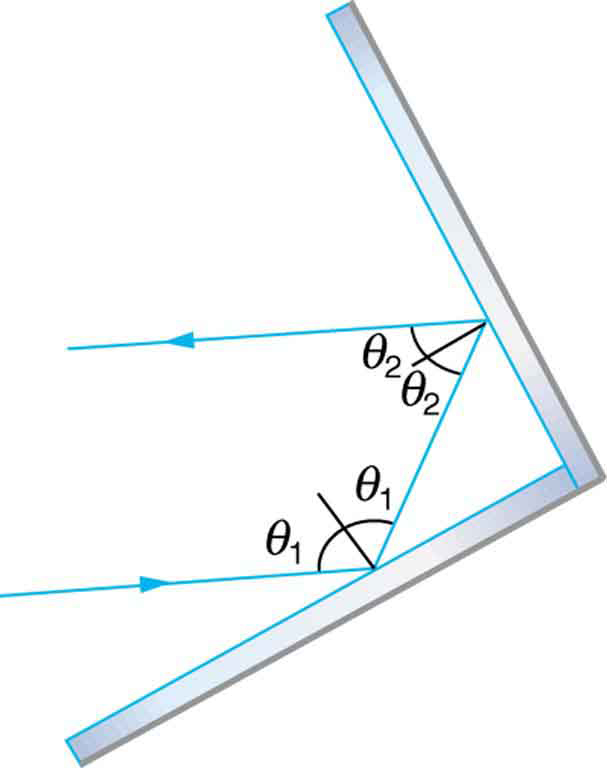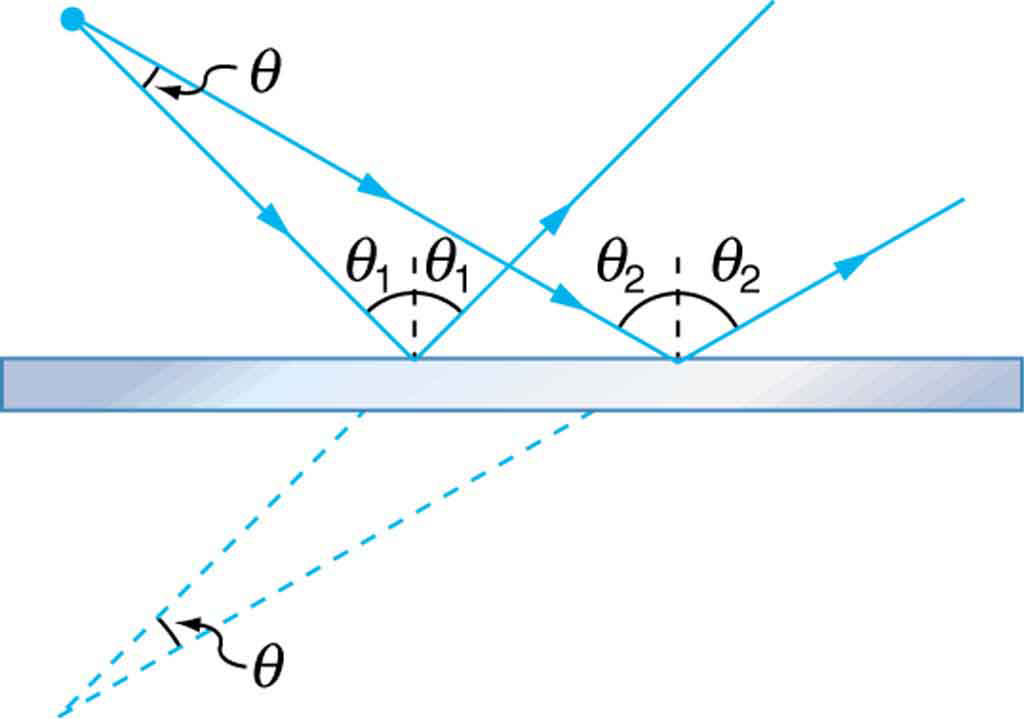# The Law of Reflection

Lumen Learning

### Learning Objectives

By the end of this section, you will be able to:

• Explain reflection of light from polished and rough surfaces.

Whenever we look into a mirror, or squint at sunlight glinting from a lake, we are seeing a reflection. When you look at this page, too, you are seeing light reflected from it. Large telescopes use reflection to form an image of stars and other astronomical objects.Figure 1. The law of reflection states that the angle of reflection equals the angle of incidence—θr = θi. The angles are measured relative to the perpendicular to the surface at the point where the ray strikes the surface.

The law of reflection is illustrated in Figure 1, which also shows how the angles are measured relative to the perpendicular to the surface at the point where the light ray strikes. We expect to see reflections from smooth surfaces, but Figure 2 illustrates how a rough surface reflects light. Since the light strikes different parts of the surface at different angles, it is reflected in many different directions, or diffused. Diffused light is what allows us to see a sheet of paper from any angle, as illustrated in Figure 3. Many objects, such as people, clothing, leaves, and walls, have rough surfaces and can be seen from all sides. A mirror, on the other hand, has a smooth surface (compared with the wavelength of light) and reflects light at specific angles, as illustrated in Figure 4. When the moon reflects from a lake, as shown in Figure 5, a combination of these effects takes place.Figure 2. Light is diffused when it reflects from a rough surface. Here many parallel rays are incident, but they are reflected at many different angles since the surface is rough.Figure 3. When a sheet of paper is illuminated with many parallel incident rays, it can be seen at many different angles, because its surface is rough and diffuses the light.Figure 4. A mirror illuminated by many parallel rays reflects them in only one direction, since its surface is very smooth. Only the observer at a particular angle will see the reflected light.Figure 5. Moonlight is spread out when it is reflected by the lake, since the surface is shiny but uneven. (credit: Diego Torres Silvestre, Flickr)

The law of reflection is very simple: The angle of reflection equals the angle of incidence.

### The Law of Reflection

The angle of reflection equals the angle of incidence.

When we see ourselves in a mirror, it appears that our image is actually behind the mirror. This is illustrated in Figure 6. We see the light coming from a direction determined by the law of reflection. The angles are such that our image is exactly the same distance behind the mirror as we stand away from the mirror. If the mirror is on the wall of a room, the images in it are all behind the mirror, which can make the room seem bigger. Although these mirror images make objects appear to be where they cannot be (like behind a solid wall), the images are not figments of our imagination. Mirror images can be photographed and videotaped by instruments and look just as they do with our eyes (optical instruments themselves). The precise manner in which images are formed by mirrors and lenses will be treated in later sections of this chapter.Figure 6. Our image in a mirror is behind the mirror. The two rays shown are those that strike the mirror at just the correct angles to be reflected into the eyes of the person. The image appears to be in the direction the rays are coming from when they enter the eyes.

### Take-Home Experiment: Law of Reflection

Take a piece of paper and shine a flashlight at an angle at the paper, as shown in Figure 3. Now shine the flashlight at a mirror at an angle. Do your observations confirm the predictions in Figure 3 and Figure 4? Shine the flashlight on various surfaces and determine whether the reflected light is diffuse or not. You can choose a shiny metallic lid of a pot or your skin. Using the mirror and flashlight, can you confirm the law of reflection? You will need to draw lines on a piece of paper showing the incident and reflected rays. (This part works even better if you use a laser pencil.)

## Section Summary

• The angle of reflection equals the angle of incidence.
• A mirror has a smooth surface and reflects light at specific angles.
• Light is diffused when it reflects from a rough surface.
• Mirror images can be photographed and videotaped by instruments.

### Conceptual Question

1. Using the law of reflection, explain how powder takes the shine off of a person’s nose. What is the name of the optical effect?

### Problems & Exercises

1. Show that when light reflects from two mirrors that meet each other at a right angle, the outgoing ray is parallel to the incoming ray, as illustrated in the following figure.Figure 7. A corner reflector sends the reflected ray back in a direction parallel to the incident ray, independent of incoming direction.
2. Light shows staged with lasers use moving mirrors to swing beams and create colorful effects. Show that a light ray reflected from a mirror changes direction by 2θ when the mirror is rotated by an angle θ.
3. A flat mirror is neither converging nor diverging. To prove this, consider two rays originating from the same point and diverging at an angle θ. Show that after striking a plane mirror, the angle between their directions remains θ.Figure 8. A flat mirror neither converges nor diverges light rays. Two rays continue to diverge at the same angle after reflection.

## Glossary

mirror: smooth surface that reflects light at specific angles, forming an image of the person or object in front of it

law of reflection: angle of reflection equals the angle of incidence